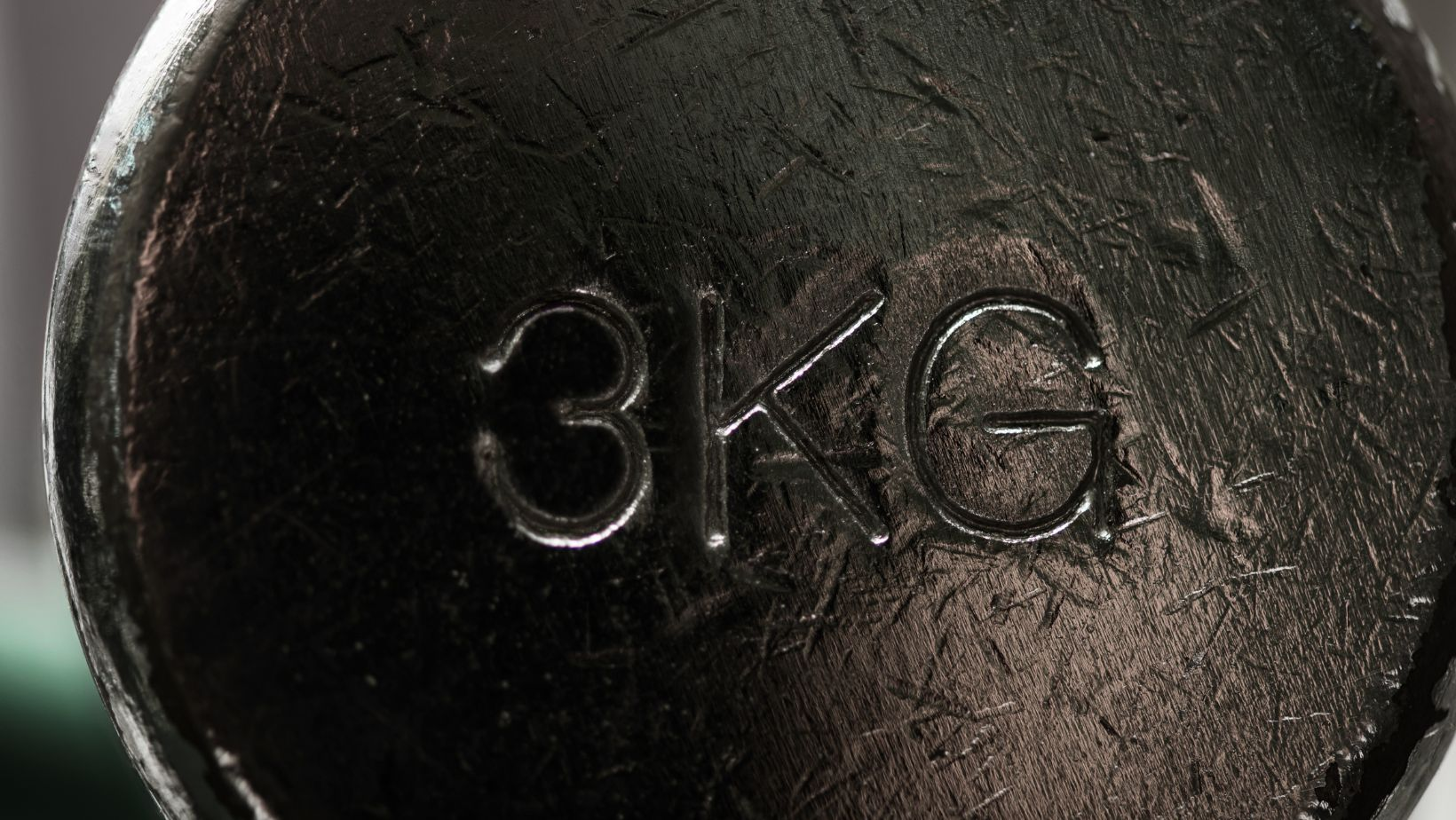# The Metric System Of How Many Ml Are in KgIf you’ve ever wondered how many milliliters are in a kilogram, you’re not alone. Many people find it perplexing to convert between units of volume and weight. But fear not! I’ll break it down for you in simple terms.

When it comes to converting kilograms to milliliters, there isn’t a direct conversion because they measure different things – weight and volume, respectively. However, if we’re talking about water, which has a density of 1 gram per milliliter (g/mL), then we can make an approximation. Since there are 1000 grams in a kilogram and the density of water is 1 g/mL, we can say that there are approximately 1000 milliliters in a kilogram of water.

## How Many Ml Are in Kg

### Calculating the Conversion Ratio

When it comes to converting milliliters (ml) to kilograms (kg), understanding the conversion ratio is key. The conversion from ml to kg depends on the substance being measured, as different substances have varying densities. Density is defined as mass divided by volume, and it helps us determine how much a given volume of a substance weighs.

To calculate the conversion ratio, we need to know the density of the substance in question. For example, if we’re working with water, which has a density of 1 gram per milliliter (g/ml), then 1 ml of water would weigh 0.001 kg (1 gram equals 0.001 kilogram). However, for other substances like oil or milk, which have different densities, the conversion ratio will be different.

### Converting Milliliters to Grams

Before converting milliliters to kilograms, it’s common practice to convert them first into grams and then into kilograms. Since there are 1,000 grams in a kilogram and 1 ml is equal to 1 gram for water at standard conditions (as mentioned earlier), this simplifies our calculations.

For instance, let’s say we have 500 ml of milk that we want to convert into kilograms. We know that milk has an approximate density of around 1.03 g/ml. So by multiplying the volume in milliliters (500 ml) by its density (1.03 g/ml), we find that our sample weighs approximately 515 grams (500 ml * 1.03 g/ml = 515 g).

### Utilizing Density for Accurate Conversions

Density plays a crucial role in accurate conversions from milliliters to kilograms. By knowing the density of a substance, we can determine the weight of a given volume and convert it accordingly.

It’s worth mentioning that densities can vary depending on factors such as temperature and pressure. Therefore, when working with substances other than water, it’s important to consider any specific conditions or variations in density that may affect your conversion calculations.

## The Basic Concept of Volume in Milliliters

### Understanding the Relationship Between Volume and Weight

When it comes to understanding the concept of volume in milliliters, it’s essential to grasp the relationship between volume and weight. Volume refers to the amount of space occupied by a substance, while weight is a measure of the force exerted on an object due to gravity. While these two concepts are related, they are not interchangeable.

In simple terms, volume is a measurement that quantifies how much space an object or substance occupies, whereas weight measures the gravitational force acting on that object or substance. For example, consider a container filled with water. The volume of water can be measured in milliliters, indicating how much space it occupies within the container. On the other hand, its weight would be measured in kilograms or pounds since it represents the force exerted by gravity on that specific mass.

### The Importance of Consistency in Measurement Units

To ensure accurate measurements and avoid confusion when converting between different units, consistency is crucial. When measuring volumes in milliliters and weights in kilograms (kg), maintaining uniformity helps prevent errors and simplifies calculations.

Using consistent units allows for easy conversion between various systems of measurement. For instance, if you have a liquid with a known density (mass per unit volume), you can convert its volume from milliliters to liters by dividing by 1000. Similarly, you can convert grams to kilograms by dividing by 1000 as well.

In conclusion,

Understanding the concept of volume in milliliters involves recognizing the relationship between volume and weight while maintaining consistency in measurement units. Exploring different conversion factors helps accurately convert between milliliters and kilograms for various substances. By grasping these fundamental concepts and utilizing appropriate conversion techniques, you can confidently navigate calculations involving volume in milliliters and its relation to weight.

Simon is an experienced cook and dedicated father who has been in the foodservice industry for over a decade. A culinary school graduate, Simon has refined and perfected his skills, both in the kitchen and at home as a father of two. He understands flavor combinations like few others do and is able to create amazing dishes with ease. In addition to his cooking skills, Simon also has the unique ability to connect with his two children. Working in kitchens around the world, he has learned how to juggle parenting duties while still finding time for himself and his family. Whether it’s reading stories with them or teaching them how to make their own meals, Simon puts a premium on teaching his children valuable life lessons that will last them well into adulthood.